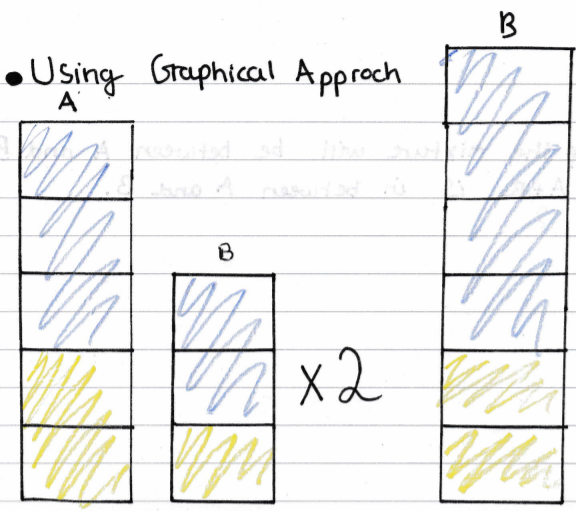#### You may also like### Tweedle Dum and Tweedle Dee

Two brothers were left some money, amounting to an exact number of pounds, to divide between them. DEE undertook the division. "But your heap is larger than mine!" cried DUM...### Sum Equals Product

The sum of the numbers 4 and 1 [1/3] is the same as the product of 4 and 1 [1/3]; that is to say 4 + 1 [1/3] = 4 � 1 [1/3]. What other numbers have the sum equal to the product and can this be so for any whole numbers?### Special Sums and Products

Find some examples of pairs of numbers such that their sum is a factor of their product. eg. 4 + 12 = 16 and 4 × 12 = 48 and 16 is a factor of 48.

##### Age 11 to 14Challenge Level

Nicholas from St. Mary & St. John, Ehab from Walton High School, Amy from Bristol Cathedral Choir School and Amy from Long Field Academy, all in the UK, all used the ratio of lemon juice to water. This is Nicholas' work:

We have 2 lemon for every 3 water for the first glass and 1 lemon and 2 water for the other. We need to make the parts that are water to the same amount. 2 and 3 both go into 6.
First glass:
3$\times$2=6 for the water now we need to multiply the lemon part by 2 as well and we get 4, so the ratio for the first glass is 4:6 lemon to water.
Second glass:
2$\times$3=6 for the water and 1$\times$3=3 for the lemon, the ratio is 3:6

The first glass has more lemon than the second
4:6 $\gt$ 3:6

Zeynep from Walton High School, Lydia from Hymers College and Louis, all in the UK, and Samaira from Prospect Primary School in Australia, used ratio/scale factors between the two lemonade mixes. This is Zeynep's work:Multiply B by 2. Then A and B will have the same amount of lemon. But B has more water. Therefore, A is the stronger tasting lemonade.

Louis explained it in a similar, but different way:

I think that the larger glass has the stronger taste because it has twice as much lemon juice as the smaller glass but only a third more water than the smaller glass. This means that the ratio of water to lemon juice is smaller which will make it taste stronger.

Samaira also used a similar but slightly different method:

Reuben from St. Mary & St. John, Jenny from Walton High School, KC from Harris Academy Battersea, Charlie, Maisy and Holly from Long Field Academy, Phoebe and Jenna from Hymers College, Ehab, Samaira and both Amys used fractions. This is Holly's work:

I first put the lemon juice as a fraction of the whole glass for each glass.
e.g.  first glass $\frac25$ and second glass  $\frac13$
I then converted the fractions so they had the same denominator
e.g. first glass $\frac6{15}$ and second glass $\frac5{15}$
I compared the numerators to see which is the highest number. This will be the one that is stronger tasting as it has a higher proportion of lemon to water. For this question the first glass will be the stronger tasting.

Amy from Long Field Academy and Joshua used percentages. Joshua wrote:

The method I used to solve this problem is making percentages. For example, if Glass A was 20 liters of lemon and 80 liters of water, then 20 percent of the mixture was lemon. Glass B might have 30 liters of lemon and 70 liters of water. 30 percent of this mixture was lemon. Whatever mixture has the larger percentage is the mixture that has the stronger lemon flavor. For example, Glass B has the stronger lemon flavor, because 30 percent of it is lemon compared to the 20 percent of Glass A.

Amy from Bristol Cathedral Choir School used decimals:

The first one has $\frac25$ lemon and $\frac35$ water. The second glass has $\frac13$ lemon and $\frac23$ water. If we convert to decimals then the first one would be $0.4$ lemon and $0.6$ water. The second one would be $0.33$ lemon and $0.67$ water.
Therefore the first glass is stronger because they both add up to one, but the first one has more lemon and less water than the second.

Amy from Bristol Cathedral Choir School looked at the difference between water and lemon juice relative to the total amount of lemonade:

After a while of looking at this problem, I realised that there was a shorter but slightly more complex way. I realised that the difference between both glasses' water and juice was 100ml: each one had 100ml more water than juice. As a proportion, the extra 100ml would matter less in a larger glass, so therefore the larger (first) glass was stronger.

Which method is better? Ehab wrote:

When the denominators are exact multiples of each other, you can make the fractions equivalent and it’s the most efficient way.

In some questions, with bigger numbers its easier to divide (for example) $200\div300$ and $100\div200$ to find which is biggest or smallest amount.

Will the strength of the combined mixture always be between the strengths of the two lemonades? Carola wrote:

Yes. Imagine lemonade A is stronger than lemonade B. To get a mixture stronger than lemonade A, you would need to have more lemon juice compared to water than lemonade A. So you would need to mix lemonade A with a lemonade that is stronger than lemonade A, not weaker. So the mixture of A and B can't be stronger than A.

To get a mixture weaker than B, you would need to mix lemonade B with a lemonade that is weaker than lemonade B, not stronger. So the mixture of A and B can't be weaker than B.

The mixture of A and B could be the same as A and B, if A and B are the same. Otherwise it will be stronger than B and weaker than A - between the two.